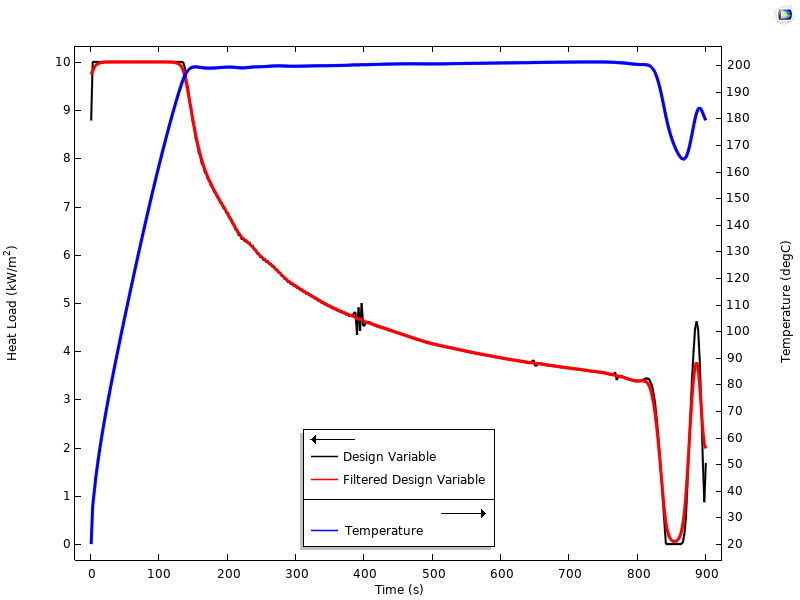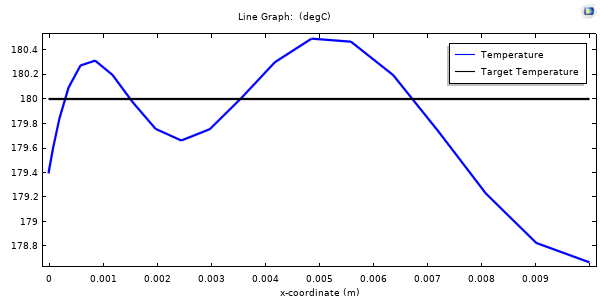# 基于方程的时间空间离散建模

2020年 9月 24日

COMSOL Multiphysics® 软件的核心优势之一是可以修改计算模型中的几乎所有表达式。我们必须谨慎使用这项功能，但是可以借助它实现其他很强大的功能。在这篇博客中，我们将以一个带有平移的二维（2D）热模型为例，先将某些材料属性设置为零，然后发现此问题变为类似于求解一维（1D）瞬态模型；最后思考这项功能是如何简便、快速地实现一些类型的优化问题。

### 将 2 个空间维度变为 1 个空间维度和 1 个时间维度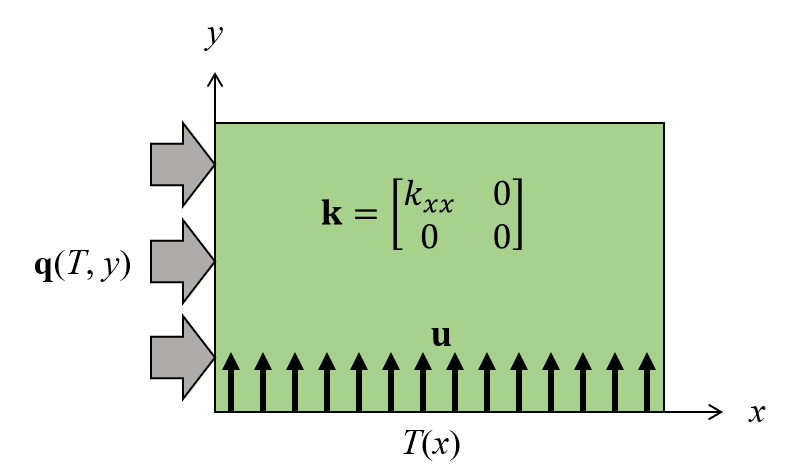\rho C_p \mathbf{u} \nabla T + \nabla \cdot \mathbf{k} \nabla T = 0

\mathbf{k}= \begin{bmatrix}k_{xx} & 0\\
0 & k_{yy}\end{bmatrix}

\rho C_p \left( u_x \frac{\partial T}
{\partial x}+ u_y \frac{\partial T}{\partial y}\right) + \frac{\partial}{\partial x}\left(k_{xx} \frac{\partial T}{\partial x}\right)+ \frac{\partial}{\partial y} \left(k_{yy} \frac{\partial T}{\partial y}\right)= 0

\rho C_p u_y \frac{\partial T}{\partial y} + \frac{\partial}{\partial x}\left(k_{xx} \frac{\partial T}{\partial x}\right) = 0

\rho C_p \frac{\partial T}{\partial t} + \frac{\partial}{\partial x}\left(k_{xx} \frac{\partial T}{\partial x}\right) = 0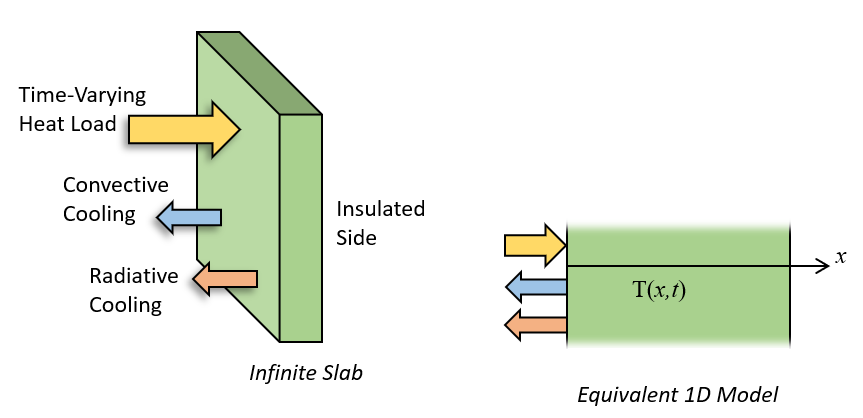### 定义优化问题

COMSOL 软件定义 节点下的拓扑优化 分支中的密度模型特征用于定义变量控制的时间热通量。这个特征在边界上定义了一个场，该场被限制在 0 到 1 之间，表示没有加热和最大加热。该场的离散化是线性的，这意味着在每个单元上热负荷随时间可以线性变化，并且热负荷可以在 ÿ 方向的网格大小确定的时间跨度上变化。这并不理想——这意味着热负荷的变化与我们的时间步一样快。相反，我们希望热负荷随时间的变化慢于离散化。因此，我们需要采取下一步骤，那就是引入亥姆霍兹过滤器（Helmholtz filter）。这在拓扑优化领域中是很常见的作法，并且可以在设置节点的过滤 部分实现。过滤半径 需要稍大于网格尺寸，并表示一个平滑的时间。该过滤后的控制变量场的名称为 dtopo1.theta，并乘以入射热通量。comp1.constr < 1

\left( \left( \frac{T}{T_{max}}\right)^{p_{exp}} \right)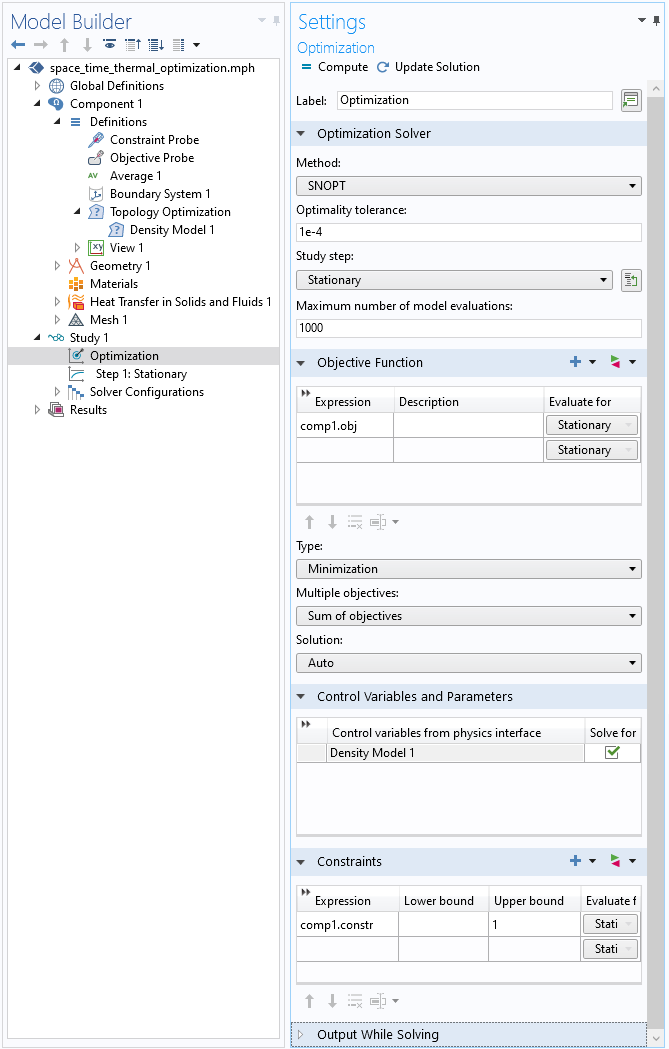“研究”分支中的“优化”研究步骤的屏幕快照，定义了优化求解器类型、目标、设计变量和约束。